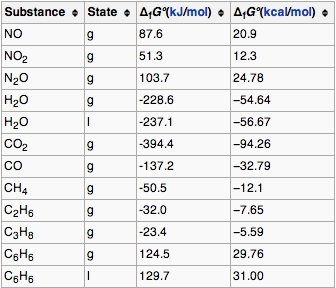## Standard Free Energy Changes

#### Learning Objective

• Calculate the change in standard free energy for a particular reaction.

#### Key Points

• The standard free energy of a substance represents the free energy change associated with the formation of the substance from the elements in their most stable forms as they exist under standard conditions.
• Combine the standard enthalpy of formation and the standard entropy of a substance to get its standard free energy of formation.
• $\Delta G^\circ (rxn) = \Sigma \Delta {G}_{f}^\circ (products) - \Sigma \Delta {G}_{f}^\circ (reactants)$ can be used to determine standard free energy change of a reaction.

#### Terms

• free energy of formationThe change of free energy that accompanies the formation of 1 mole of a substance in its standard state from its constituent elements in their standard states.
• entropyA thermodynamic property that is the measure of a system’s thermal energy per unit temperature that is unavailable for doing useful work.
• enthalpyIn thermodynamics, a measure of the heat content of a chemical or physical system.

## Reviewing Standard States

The concept of standard states is especially important in the case of free energy, so take a moment to review it. For most practical purposes, the following definitions of standard states are acceptable:

• Gases: 1 atmosphere partial pressure.
• Pure liquids: the liquid under a total pressure of 1 atm.
• Solutes: an effective concentration of 1 Molar.
• Solids: the pure solid under 1 atm pressure.

Note also that there is actually no “standard temperature”, but because most thermodynamics tables list values for 298.15 K (25° C), this temperature is usually implied. These same definitions apply to standard enthalpies and internal energies. Don’t confuse these thermodynamic standard states with the “standard temperature and pressure” (STP) widely employed in gas law calculations.

## Calculating Gibbs Free Energy

In order to make use of Gibbs energies to predict chemical changes, it is necessary to know the free energies of the individual components of the reaction. To accomplish this, combine the standard enthalpy and the standard entropy of a substance to get the standard free energy of a reaction:

$\Delta {G}^\circ= \Delta {H}^\circ-T\Delta {S}^\circ$

Recall that the symbol ° refers to the standard state of a substance measured under the conditions of 1 atm pressure or an effective concentration of 1 Molar and a temperature of 298K. The other factor to keep in mind is that enthalpy values are normally given in $\frac{kJ}{mole}$ while entropy values are given in $\frac{J}{K\times mole}$ . The energy units will need to be the same in order to solve the equation properly.

The standard Gibbs free energy of the reaction can also be determined according to:

$\Delta G^\circ (rxn)= \Sigma \Delta {G}_{f}^\circ(products)-\Sigma \Delta {G}_{f}^\circ(reactants)$

As with standard heats of formation, the standard free energy of a substance represents the free energy change associated with the formation of the substance from the elements in their most stable forms as they exist under the standard conditions of 1 atm pressure and 298K. Standard Gibbs free energies of formation are normally found directly from tables. Once the values for all the reactants and products are known, the standard Gibbs free energy change for the reaction can be found. Most tables of thermodynamic values list ΔGf°’s for common substances. They can, of course, always be found from values of $\Delta {H}_{f}^\circ$ and $\Delta {S}_{f}^\circ$ .Gibbs Energy of FormationThe standard Gibbs free energy of formation of a compound is the change of Gibbs free energy that accompanies the formation of 1 mole of that substance from its component elements, at their standard states.

Example Problem: Calculate the $\Delta G^0_{rxn}$ for the following equation using the values in the table:

$CO_{(g)} + \frac {1}{2} O_{2(g)} \rightarrow CO_{2(g)}$

$\Delta G^\circ (rxn)= \Sigma \Delta {G}_{f}^\circ(products)-\Sigma \Delta {G}_{f}^\circ(reactants)$

*Remember that substances in elemental form (such as O2) have $\Delta G^o_f$ values equal to zero. It is also important to remember that the table provides per mole values. If the balanced equation calls for more than one mole the $\Delta G^o_f$ must be multiplied.

$\Delta G^\circ (rxn)= (-394.4\ kJ) -(-137.2\ kJ + 0\ kJ)$

$\Delta G^\circ (rxn)= -257.2\ kJ$